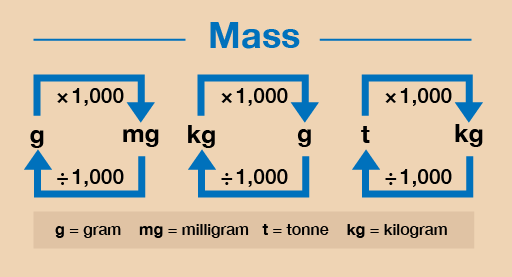Everyday maths 1 (Wales)

Start this free course now. Just create an account and sign in. Enrol and complete the course for a free statement of participation or digital badge if available.

Free course

# 3.3 Converting metric units of weight

There are occasions where you may have to convert between metric units of weight. Figure 25 shows you how to do this. In this section, we are only going to practise converting between grams (g) and kilograms (kg).

Hint: Weight is sometimes referred to as mass.Figure 25 A conversion chart for weight

## Example: Converting units of weight

1. Convert the following from kilograms into grams:
• a.4 kg = ? g
• b.6.5 kg = ? g
2. Convert the following from grams into kilograms:
• a.8 000 g = ? kg
• b.1 250 g = ? kg

### Method

1. As you can see from Figure 25, to convert from kilograms (kg) to grams (g) you need to multiply by 1 000:
• a.4 kg × 1 000 = 4 000 g
• b.6.5 kg × 1 000 = 6 500 g
2. If you want to convert from grams (g) to kilograms (kg) you need to divide by 1 000:
• a.8 000 g ÷ 1 000 = 8 kg
• b.1 250 g ÷ 1 000 = 1.25 kg

Now try the following activity.

## Activity 13: Converting metric units of weight

Calculate the following without using a calculator. You may wish to look back at Session 1 to remind you how to multiply [Tip: hold Ctrl and click a link to open it in a new tab. (Hide tip)] and divide by 1 000.

You may double-check your answer with a calculator if you need to. Remember to check your answers.

1. Convert the following to kilograms:
• a.3 000 g
• b.9 500 g
• c.750 g
• d.10 000 g
2. Convert the following to grams:
• a.4 kg
• b.1.5 kg
• c.7.6 kg
• d.2.25 kg

### Answer

1. The answers are as follows:
• a.3 000 g ÷ 1 000 = 3 kg
• b.9 500 g ÷ 1 000 = 9.5 kg
• c.750 g ÷ 1 000 = 0.75 kg
• d.10 000 g ÷ 1 000 = 10 kg
2. The answers are as follows:
• a.4 kg × 1 000 = 4 000 g
• b.1.5 kg × 1 000 = 1 500 g
• c.7.6 kg × 1 000 = 7 600 g
• d.2.25 kg × 1 000 = 2 250 g
FSM_1_CYMRU

### Take your learning further

Making the decision to study can be a big step, which is why you'll want a trusted University. The Open University has 50 years’ experience delivering flexible learning and 170,000 students are studying with us right now. Take a look at all Open University courses.

If you are new to University-level study, we offer two introductory routes to our qualifications. You could either choose to start with an Access module, or a module which allows you to count your previous learning towards an Open University qualification. Read our guide on Where to take your learning next for more information.

Not ready for formal University study? Then browse over 1000 free courses on OpenLearn and sign up to our newsletter to hear about new free courses as they are released.

Every year, thousands of students decide to study with The Open University. With over 120 qualifications, we’ve got the right course for you.

Request an Open University prospectus371